Ex 13.3

Chapter 13 Class 10 Surface Areas and Volumes
Serial order wise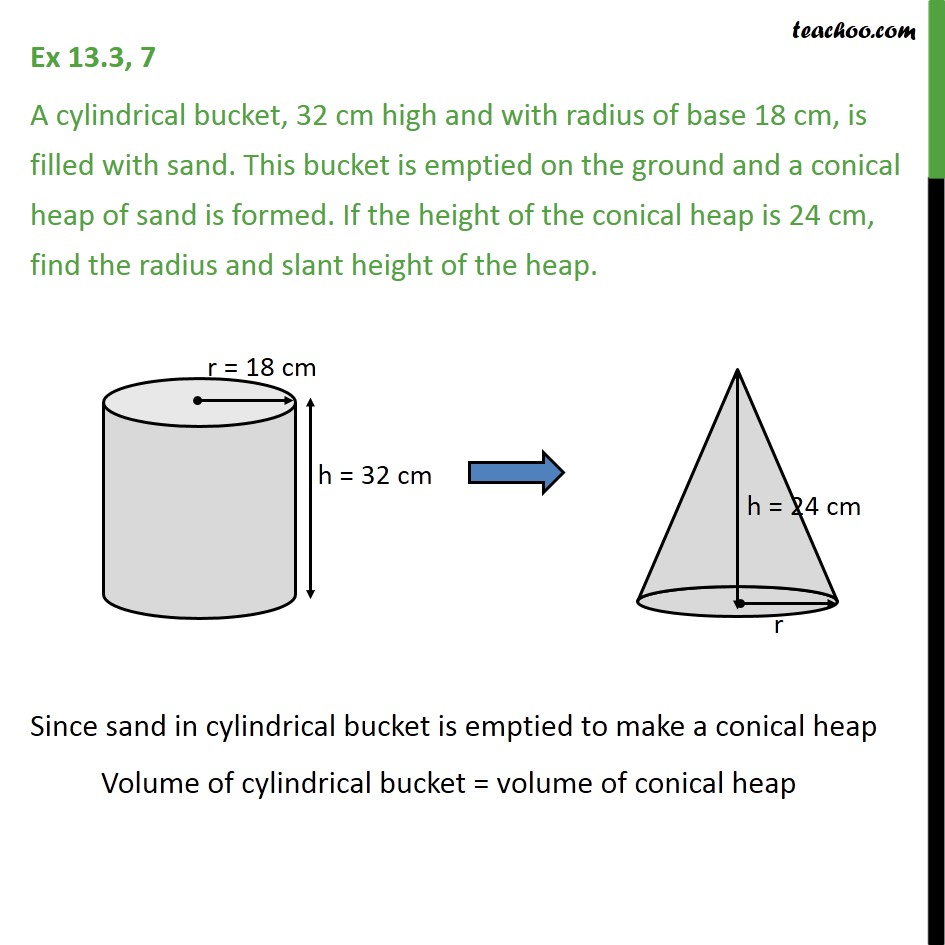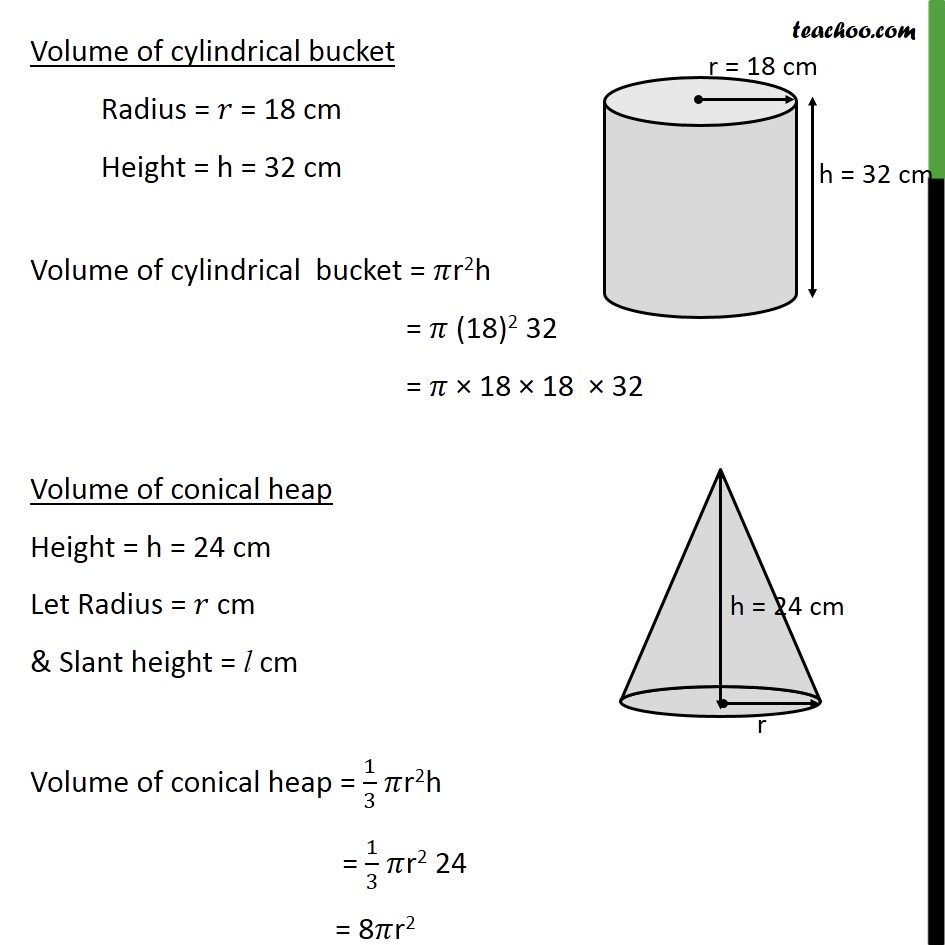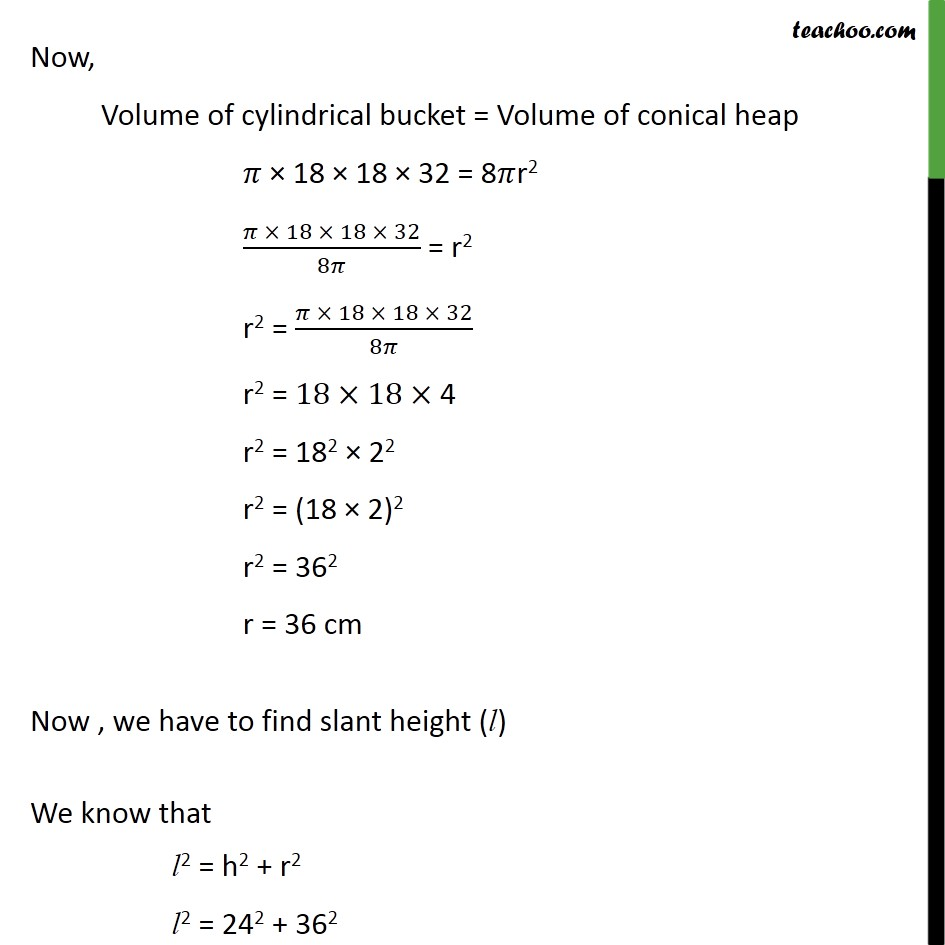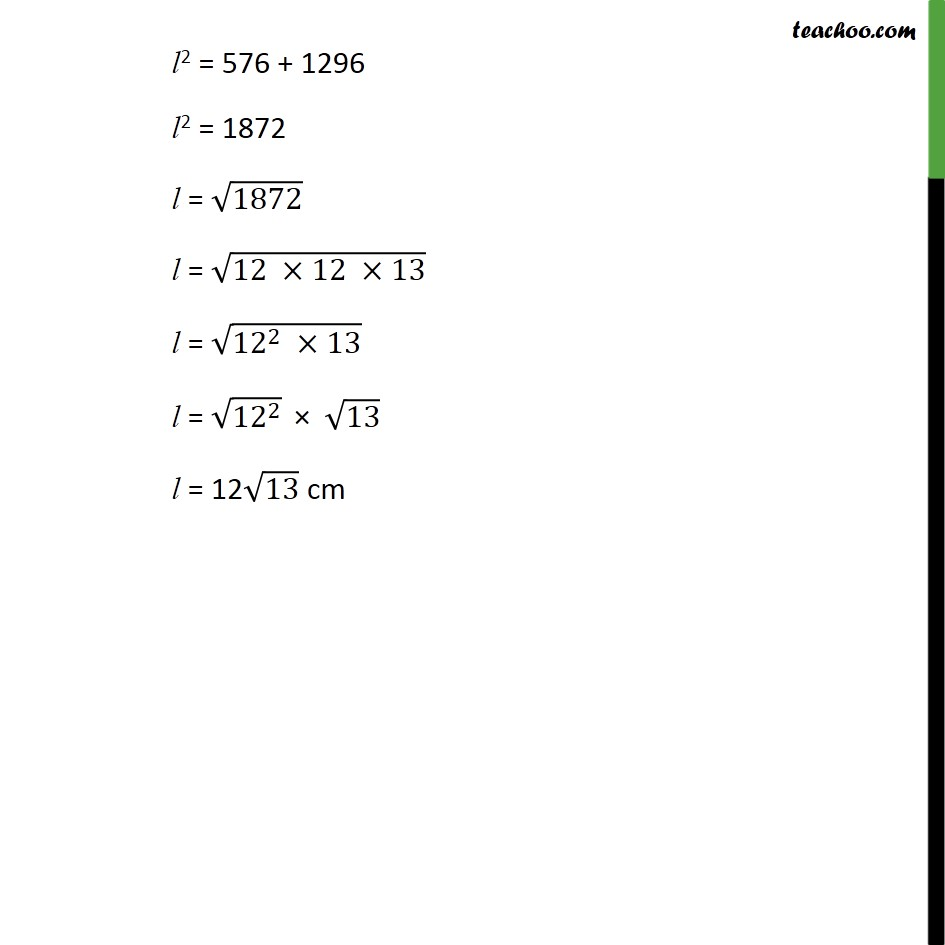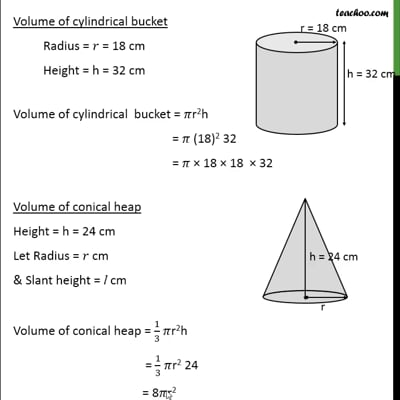This video is only available for Teachoo black users

Solve all your doubts with Teachoo Black (new monthly pack available now!)

### Transcript

Ex 13.3, 7 A cylindrical bucket, 32 cm high and with radius of base 18 cm, is filled with sand. This bucket is emptied on the ground and a conical heap of sand is formed. If the height of the conical heap is 24 cm, find the radius and slant height of the heap. Since sand in cylindrical bucket is emptied to make a conical heap Volume of cylindrical bucket = volume of conical heap Volume of cylindrical bucket Radius = 𝑟 = 18 cm Height = h = 32 cm Volume of cylindrical bucket = 𝜋r2h = 𝜋 (18)2 32 = 𝜋 × 18 × 18 × 32 Volume of conical heap Height = h = 24 cm Let Radius = 𝑟 cm & Slant height = l cm Volume of conical heap = 1/3 𝜋r2h = 1/3 𝜋r2 24 = 8𝜋r2 Now, Volume of cylindrical bucket = Volume of conical heap 𝜋 × 18 × 18 × 32 = 8𝜋r2 (𝜋 × 18 × 18 × 32)/8𝜋 = r2 r2 = (𝜋 × 18 × 18 × 32)/8𝜋 r2 = 18×18× 4 r2 = 182 × 22 r2 = (18 × 2)2 r2 = 362 r = 36 cm Now , we have to find slant height (l) We know that l2 = h2 + r2 l2 = 242 + 362 l2 = 576 + 1296 l2 = 1872 l = √1872 l = √(12 ×12 ×13) l = √(〖12〗^2 ×13) l = √(〖12〗^2 ) × √13 l = 12√13 cm